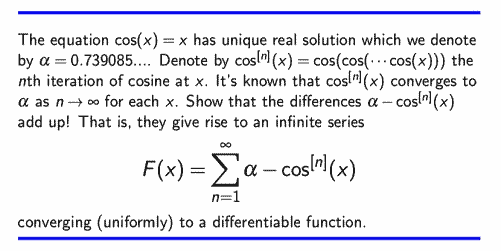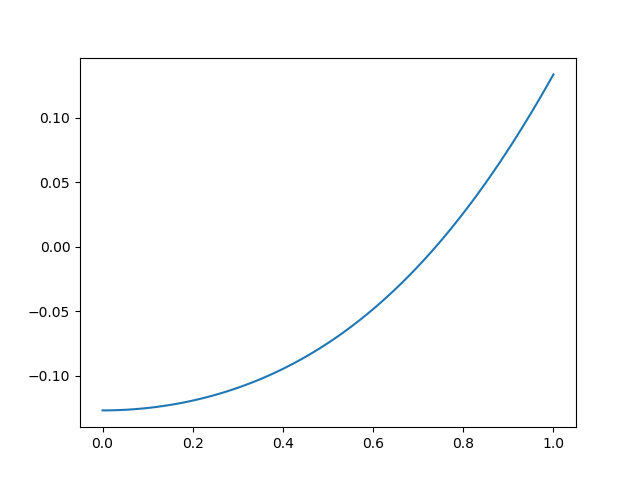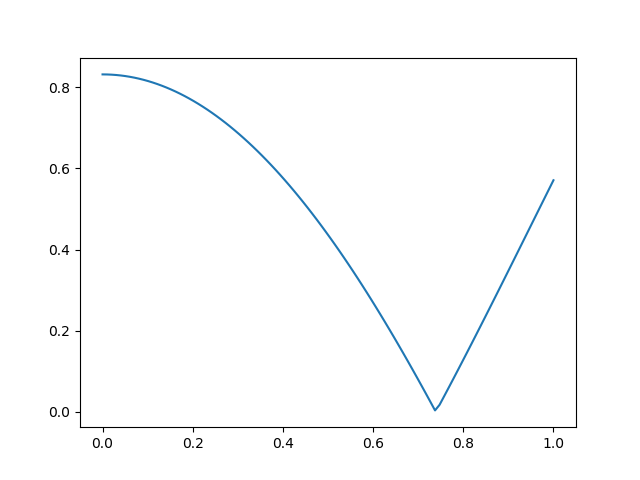# Variation on cosine fixed point

If you enter any number into a calculator and repeatedly press the cosine key, you’ll eventually get 0.73908513, assuming your calculator is in radian mode . And once you get this value, taking the cosine more times won’t change the number. This is the first example of a fixed point of an iteration that many people see.

Sam Walter posted a variation on the cosine fixed point problem on Twitter recently. If you add up the distance from each iteration value to the fixed point, the sum converges. Furthermore, if you consider this sum as a function of the starting point x, it defines a differentiable function of x.I was curious what this function looks like, so I wrote the following Python code. First we find the fixed point.

```    from math import cos

epsilon = 1e-16
x, cx = 1, cos(1)
while abs(x - cx) > epsilon:
x, cx = cx, cos(cx)

alpha = x # fixed point
```

Now let’s evaluate the function described above.

```    def f(x):
s = 0
cx = cos(x)
delta = alpha - cx
while abs(delta) > epsilon:
s += delta
x, cx = cx, cos(cx)
delta = alpha - cx
return s
```

Here’s what the plot of our function looks like.As we’d expect, it crosses the x-axis at the fixed point.

If we sum the absolute distance to the fixed point at each iteration, we get the following function with a minimum at the fixed point.## Python’s new walrus operator

Incidentally, we can make the code slightly more compact if we take advantage of a new feature in Python 3.8, affectionately known as the “walrus operator.” The `:=` operator lets you do assignments inside a loop test, among other places, much like is commonly done in C.

```    def f(x):
s, cx = 0, cos(x)
while abs(delta := alpha - cx) > 1e-16:
s += delta
x, cx = cx, cos(cx)
return s
```

Now we don’t need to compute `delta` before the loop, and we can update delta and check its absolute value in the same place.

I’m not sure how I feel about the walrus operator. It could make code easier to read or harder to read, depending on context.

One advantage of Python’s walrus over its C analog is that it prevents bugs due to typing `=` when you mean to type `==`. If you really want to do an assignment inside a Boolean test, you’ll need an extra character, the colon in front of the equal sign; using an ordinary equal sign alone is a syntax error.

## More fixed point posts

 If your calculator is in degree mode, you’ll still get a fixed point, but the fixed point will be 0.99984774 degrees. Note that this is not simply the fixed point for cosine of an angle in radians, converted to degrees. Cosine of x degrees is a different function than cosine of x radians and it has a different fixed point.